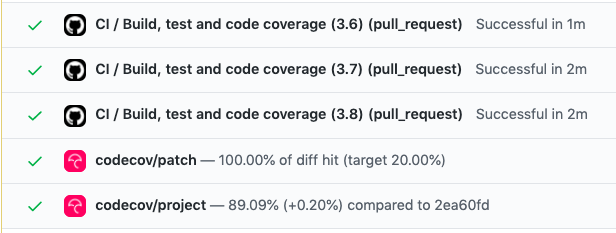Hey there! Welcome to the second blog in the series of GSoC blogs. I’ll be writing about the developments made over the past couple of weeks.

### GitHub Actions

I have successfully set up GitHub actions to do automated testing and code coverage.### Pull up vs push down

The way testbook was headed initially followed a more pull up approach where cell outputs would be “pulled up” from the Jupyter kernel into the unit test upon which assertions will be performed.

However, this was very restricting because non-JSON serializeable objects would not be able to be pulled up. After discussing this with my mentor Matthew Seal, we realized that it would be best to push down the assertions instead.

Soon after that, Matt and I had a pair programming session where he suggested some ways in which we could push down the assertions - by creating reference objects that would reference variables/functions in the kernel.

This set me off on the right path and I was able to come up with an implementation that looks something like this:

``````class TestbookObjectReference:
def __init__(self, tb, name):
self.tb: TestbookNotebookClient = tb
self.name: str = name

def __getattr__(self, name):
return TestbookObjectReference(self.tb, f"{self.name}.{name}")

def __eq__(self, rhs):
return self.tb._assert_in_notebook(self.name, rhs)

def __call__(self, *args, **kwargs):
code = self.tb._construct_call_code(self.name, args, kwargs)
return self.tb.value(code)

def resolve(self):
return self.tb.value(self.name, safe=False)
``````

With this, it would be easy to reference objects in the kernel without needing to pull up their value.

The main thing in the code above is the `__eq__` method which is what makes assertions happen in the kernel.

#### And now, a simple unit test can be written as follows

``````from testbook import testbook
import pytest

@pytest.mark.parametrize("a, b, expected", [
(
(1, 0), (0, 1), 2 ** 0.5
),
(
(1, 0), (0, 0), 1
),
])
def test_calculate_distance(a, b, expected):
with testbook('notebook.ipynb', execute=True) as tb:
calculate_distance = tb.ref("calculate_distance") # returns a reference

assert calculate_distance(a, b) == expected
``````